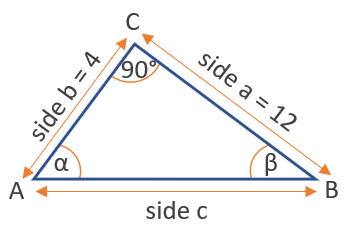# Arccot Calculator

Use this arccot calculator to easily calculate the arccotangent of a given number. Supports input of either decimal numbers (e.g. 0.5, -0.5) or fractions (e.g. 1/2, -1/2).

### Calculation results

63.434949 degrees arccot(0.5) = arccot(0.5) =
Share calculator:

Embed this tool:
get code

## Arccot function

The arccot is one of the inverse trigonometric functions (antitrigonometric functions) and is the inverse of the cotangent function. It is sometimes written as cot-1(x), but this notation should be avoided as it might be confused with an exponent notation. The arccot is used to obtain an angle from the cotangent trigonometric ratio, which is the ratio between the side adjacent to the angle and the side opposite to it.

The function spans all real numbers (-∞ - +∞) and so do the results from our arccot calculator. The range of the angle values is usually between 0° and 180°. There are a number of arccot rules, like that tan(arccot(x)) = 1/x, that or sin(arccot(x)) = 1 / √(1+x2), or that cos(arccot(x)) = x / √(1+x2), which can help you in trigonometry calculus.

## How to calculate the arccot of a number?

The easiest way to calculate it is by using our arccot calculator above, which will output results in both degrees and radians. Other ways include other given information, such as the values of other trigonometric functions for the same angle or other angles in the same triangle.

Here is a table of common arccot values:

Common values of the arccot function
-∞ 180° π
-√3 150° 5π/6
-1 135° 3π/4
-1/√3 120° 2π/3
0 90° π/2
1/√3 60° π/3
1 45° π/4
√3 30° π/5
+∞ 0

π is, of course, the mathematical constant about equal to 3.14159.

## Example: find an angle using arccot

Assume there is need to know the angle at which two lines meet at point A, but we only have a ruler with a straight angle on it to work with. We can assess that the angle at point C is right (90 degrees) and measure the lengths of side a = 12 and side b = 4. How do we find the angle α at point A without taking additional measures?First, calculate the cotangent of α by dividng the opposite by the hypotenuse. This way cot(α) = b / a = 4 / 12 = 0.333 can be computed. Then use the inverse cotangent function arccot with this outcome to calculate the angle α = arccot(0.333) = 71.58° (1.25 radians).

#### Cite this calculator & page

If you'd like to cite this online calculator resource and information as provided on the page, you can use the following citation:
Georgiev G.Z., "Arccot Calculator", [online] Available at: https://www.gigacalculator.com/calculators/arccot-calculator.php URL [Accessed Date: 01 Apr, 2023].